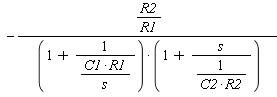# Rearranging equation into another form.

## Homework Statement

I have a transfer function as part of a circuit analysis question which I obtained as:

$-{\frac {{\it R2}}{ \left( {\it sC1}\,{\it R1}+1 \right) \left( {\it R2}\,{\it sC2}+1 \right) }}$

Which needs to be turned into:## The Attempt at a Solution

I have attempted by multiplying the top and bottom of the fraction by R1, s, C1, etc, trying to manipulate to get into the required form, but no luck yet.

Are C's and R's related in any way?

Suppose you set C1 = 0. Then the first expression is finite whereas the second one is zero.

Are C's and R's related in any way?

Suppose you set C1 = 0. Then the first expression is finite whereas the second one is zero.

C stands for the capacitance, and R is the resistance.

I guess I should have said C and R are non-zero. I would put the circuit diagram, but it wouldn't make sense unless you can understand it, although it's pretty easy.

I'm not sure what you mean by are they related. It's like having an equation in x and y.